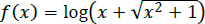## Aspire's Library

A Place for Latest Exam wise Questions, Videos, Previous Year Papers,
Study Stuff for MCA Examinations

## NIMCET Previous Year Questions (PYQs)

#### NIMCET Function PYQ

NIMCET PYQ
The graph of function $f(x)=\log _e({x}^3+\sqrt[]{{x}^6+1})$ is symmetric about:

NIMCET Previous Year PYQNIMCET NIMCET 2023 PYQ

#### Solution

NIMCET PYQ
If f(x) is a polynomial of degree 4, f(n) = n + 1 & f(0) = 25, then find f(5) = ?

NIMCET Previous Year PYQNIMCET NIMCET 2023 PYQ

#### Solution

NIMCET PYQ
The maximum value of $f(x) = (x – 1)^2 (x + 1)^3$ is equal to $\frac{2^p3^q}{3125}$  then the ordered pair of (p, q) will be

NIMCET Previous Year PYQNIMCET NIMCET 2023 PYQ

#### Solution

NIMCET PYQ
If $| x - 6|= | x - 4x | -| x^2- 5x +6 |$ , where x is a real variable

NIMCET Previous Year PYQNIMCET NIMCET 2023 PYQ

#### Solution

NIMCET PYQ
A real valued function f is defined as $f(x)=\begin{cases}{-1} & {-2\leq x\leq0} \\ {x-1} & {0\leq x\leq2}\end{cases}$.  Which of the following statement is FALSE?

NIMCET Previous Year PYQNIMCET NIMCET 2023 PYQ

#### Solution

NIMCET PYQ
Number of onto (surjective) functions from A to B if n(A)=6 and n(B)=3, is

NIMCET Previous Year PYQNIMCET NIMCET 2019 PYQ

#### Solution

NIMCET PYQ
Let $X_i, i = 1,2,.. , n$ be n observations and $w_i = px_i +k, i = 1,2, ,n$ where p and k are constants. If the mean of $x_i 's$ is 48 and the standard deviation is 12, whereas the mean of $w_i 's$ is 55 and the standard deviation is 15, then the value of p and k should be

NIMCET Previous Year PYQNIMCET NIMCET 2019 PYQ

#### Solution

NIMCET PYQ
Let S be the set $\{a\in Z^+:a\leq100\}$.If the equation $[tan^2 x]-tan x - a = 0$ has real roots (where [ . ] is the greatest integer function), then the number of elements is S is

NIMCET Previous Year PYQNIMCET NIMCET 2019 PYQ

#### Solution

NIMCET PYQ
The domain of the function $f(x)=\frac{{\cos }^{-1}x}{[x]}$ is

NIMCET Previous Year PYQNIMCET NIMCET 2022 PYQ

#### Solution

NIMCET PYQ
The function $f(x)=\log (x+\sqrt[]{{x}^2+1})$ is

NIMCET Previous Year PYQNIMCET NIMCET 2022 PYQ

#### Solution

NIMCET PYQ
The functionis

NIMCET Previous Year PYQNIMCET NIMCET 2018 PYQ

#### Solution

NIMCET PYQ
Which of the following function is the inverse of itself?

NIMCET Previous Year PYQNIMCET NIMCET 2018 PYQ

#### Solution

NIMCET PYQ
If the graph of y = (x – 2)2 – 3 is shifted by 5 units up along y-axis and 2 units to the right along the x-axis, then the equation of the resultant graph is

NIMCET Previous Year PYQNIMCET NIMCET 2017 PYQ

#### Solution

When y= f (x) is shifted by k units to the right along x
– axis, it become y= f (x - k )
Hence, new equation of
graph is y = (x - 4)2 + 2

#### NIMCET

Online Test Series,
and More.

#### NIMCET

Online Test Series,
and More.